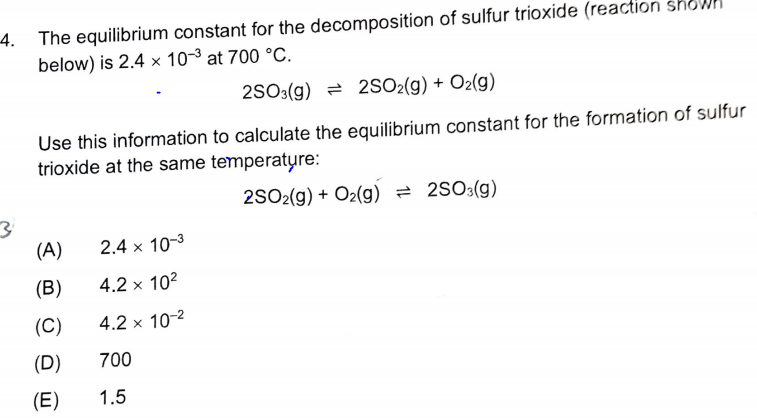# The equilibrium constant for the decomposition of sulfur trioxide (reaction shown below) is 2.4x10^-3 at 700 C. 2 SO3(g) ⇌ 2 SO2(g) + O2(g) Use this information to calculate the equilibrium constant for the formation of sulfur trioxide at the same temperature: 2 SO2(g) + O2(g) ⇌ 2 SO3(g)